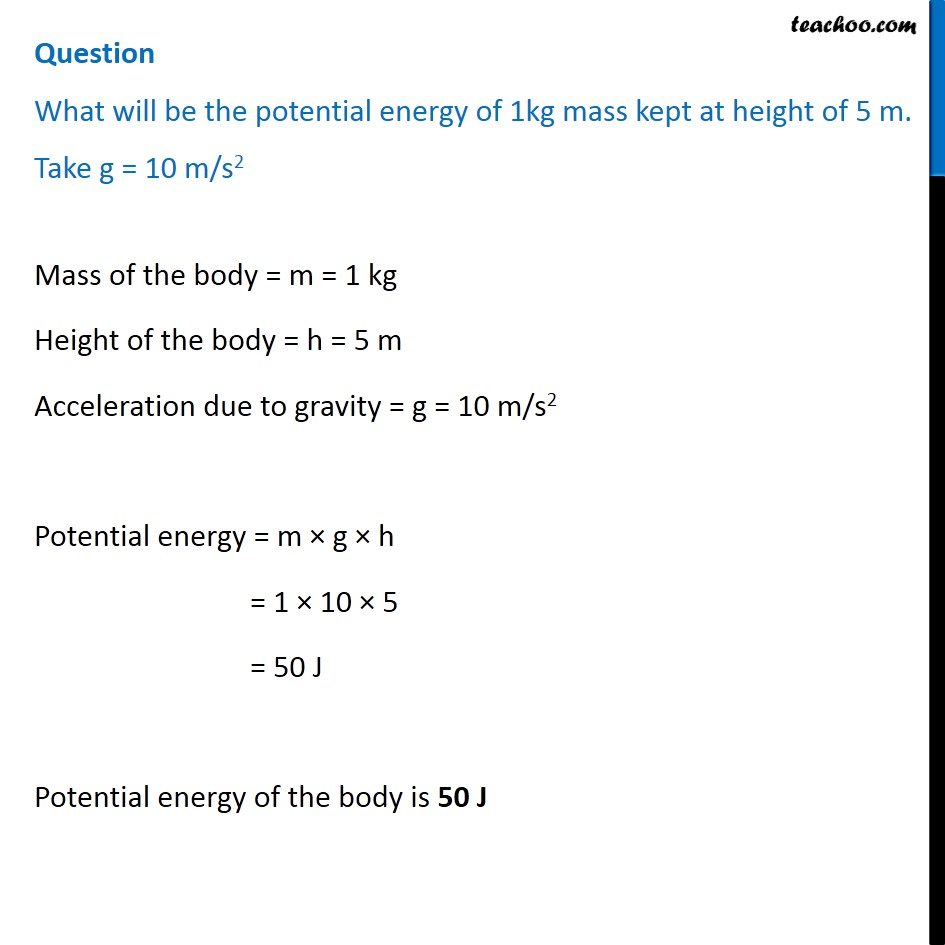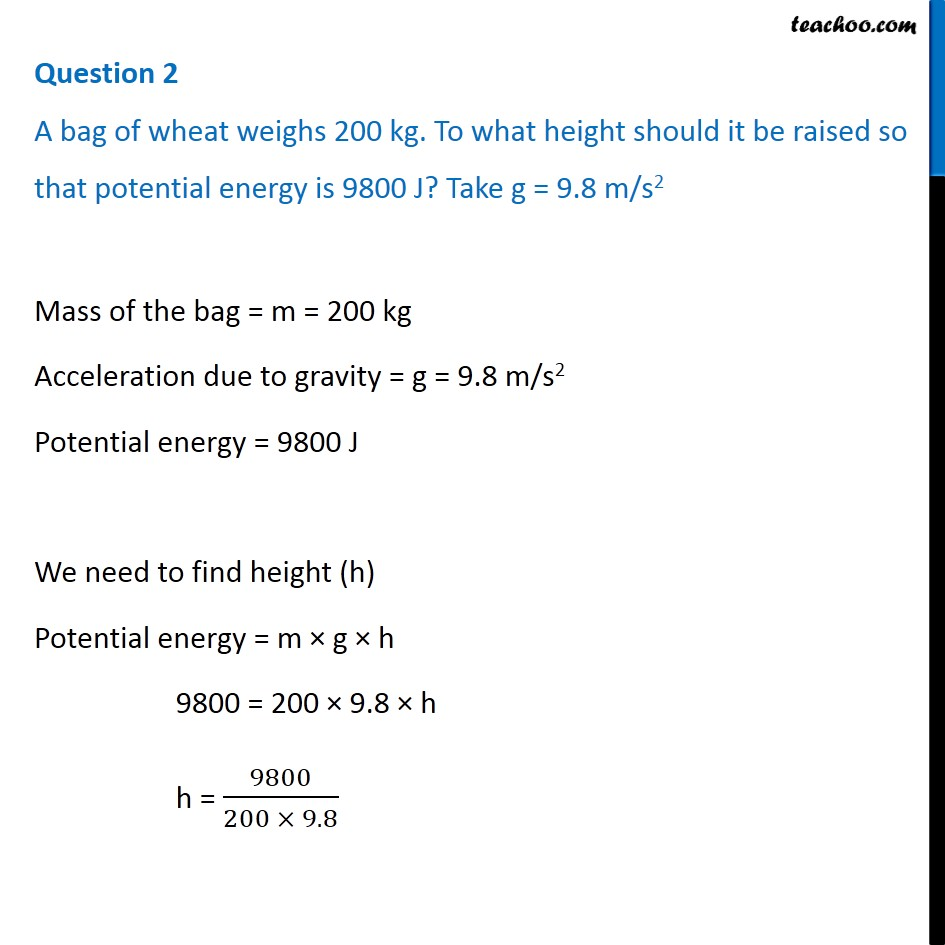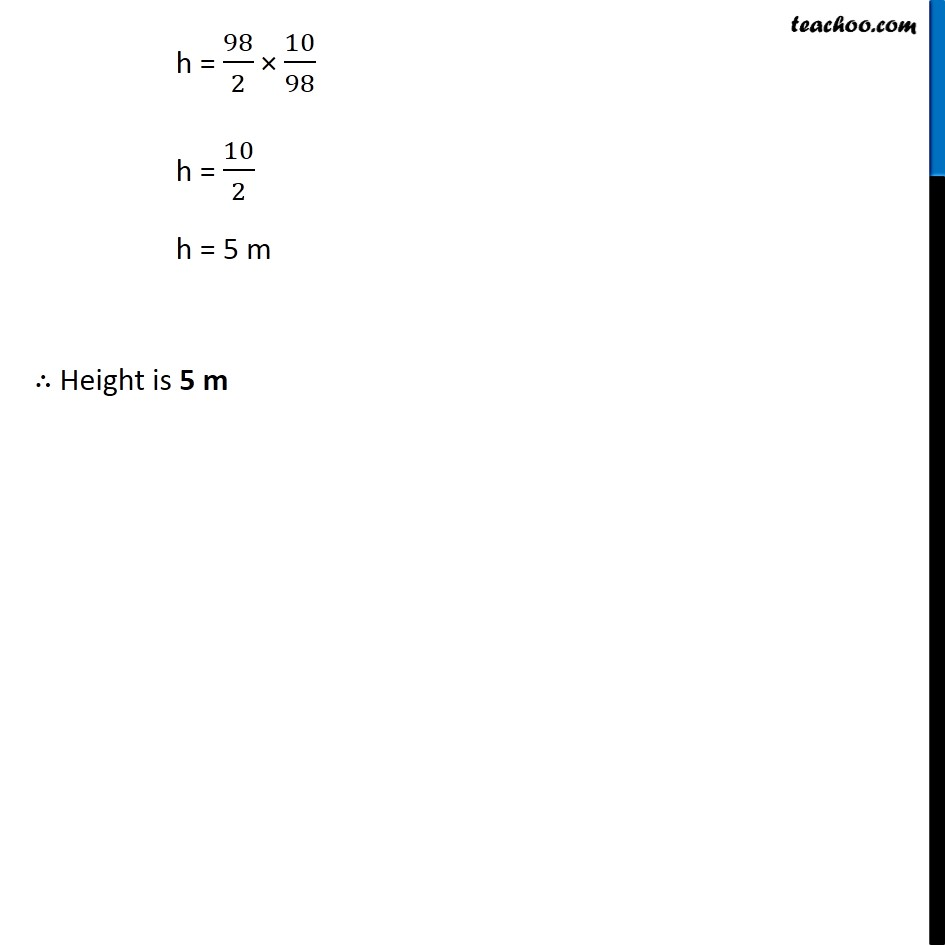Concepts

Class 9
Chapter 11 Class 9 - Work and Energy

## What is Meaning of Potential

Potential means having the capacity to do something

Example

A child has potential to score good marks in exams

A brick has potential to hurt us

## What is Potential Energy

Energy stored in a body because of its position or change in shape is called Potential Energy

Example 1

brick lying at ground does not have potential energy

But if we pick up a brick it has potential energy

Example 2

An arrow attached to bow has no potential energy

But if we stretch the bow, it has potential energy## Different Types of Potential Energy

This energy is of 2 type

### Gravitational Potential Energy-

Potential Energy due to position of an object

Example - A raised brick, Brick lying on terrace

### Elastic Potential Energy-

Potential Energy due to change in shape

Example - Stretched Rubber String, Stretched bow

## How does a raised brick have Potential Energy?

Suppose brick is lying at ground

It currently has no potential energy

We pick it up applying our muscular energy

We position it towards our feet

At this point of time,we have spent energy in picking up the brick

This energy is stored in the brick in the form of potential energy

(If we let go the brick at this point of time,it has the potential to hurt our feet)

Hence,we say that brick has potential energy because of its relative position compared to our feet

## Potential and Kinetic Energy in birds

A bird sitting on a tree branch has potential energy

But when it flies, it has both potential and kinetic energy

Note-Sum of Potential and Kinetic Energy is called Mechanical Energy

## How to Calculate Gravitational Potential EnergyWe know that Gravitational Potential Energy is due to Position of body lying at a height above earth

Potential Energy is equal to Work done by body in moving against gravity to reach at that height

So

Potential Energy = Work Done in Moving against gravity

Potential Energy = Force × Distance

Potential Energy = Mass × Acceleration × Distance

Potential Energy = Mass × Acceleration due to gravity × Height

Potential Energy = m × g × h

## Questions## Questions

Example 11.5 - Find the energy possessed by an object of mass 10 kg when it is at a height of 6 m above the ground. Given, g = 9.8 m s –2.

Example 11.6 - An object of mass 12 kg is at a certain height above the ground. If the potential energy of the object is 480 J, find the height at which the object is with respect to the ground. Given, g = 10 m s –2 .

Learn in your speed, with individual attention - Teachoo Maths 1-on-1 Class

### Transcript

Question What will be the potential energy of 1kg mass kept at height of 5 m. Take g = 10 m/s2 Mass of the body = m = 1 kg Height of the body = h = 5 m Acceleration due to gravity = g = 10 m/s2 Potential energy = m × g × h = 1 × 10 × 5 = 50 J Potential energy of the body is 50 J Question 2 A bag of wheat weighs 200 kg. To what height should it be raised so that potential energy is 9800 J? Take g = 9.8 m/s2 Mass of the bag = m = 200 kg Acceleration due to gravity = g = 9.8 m/s2 Potential energy = 9800 J We need to find height (h) Potential energy = m × g × h 9800 = 200 × 9.8 × h h = 9800/(200 × 9.8) h = 98/2 × 10/98 h = 10/2 h = 5 m ∴ Height is 5 m